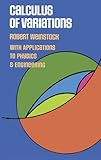» » Barcode: 0000486630692Tag# Calculus of Variations: with Applications to Physics and Engineering( Book Product )Rank: 15%Calculus of Variations: with Applications to Physics and Engineering

Specifications

• Product weight is 0.82 lbs.
This book by Robert Weinstock was written to fill the need for a basic introduction to the calculus of variations. Simply and easily written, with an emphasis on the applications of this calculus, it has long been a standard reference of physicists, engineers, and applied mathematicians.The author begins slowly, introducing the reader to the calculus of variations, and supplying lists of essential formulae and derivations. Later chapters cover isoperimetric problems, geometrical optics, Fermat's principle, dynamics of particles, the Sturm-Liouville eigenvalue-eigenfunction problem, the theory of elasticity, quantum mechanics, and electrostatics. Each chapter ends with a series of exercises which should prove very useful in determining whether the material in that chapter has been thoroughly grasped.The clarity of exposition makes this book easily accessible to anyone who has mastered first-year calculus with some exposure to ordinary differential equations. Physicists and engineers who find variational methods evasive at times will find this book particularly helpful."I regard this as a very useful book which I shall refer to frequently in the future." J. L. Synge, Bulletin of the American Mathematical Society.

References
^ (1974). Calculus of Variations: with Applications to Physics and Engineering, Dover Publications. Amazon. (revised Apr 2015)

 Page 1 of 1 1
 Page 1 of 1 1

## Calculus of Variations: with Applications to Physics and Engineering (2019-01-06)

### Account

Social:
Pages:  ..   ..
Items:  ..

Help:  ..   ..
Category:  ..   ..
Media:  ..   ..
Posts:  ..   ..   ..

### Statistics

Page:  ..
Summary:  ..
1 Tags
2/10 Page Rank
10 Page Refs
3s Time
32 Sources